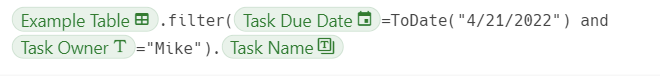Share
Explore
Hello, Database!

#Comparison of a simple formula in Sheets vs CodaComparison of a simple formula in Sheets vs Coda

Example Table
0
1
Suzie
4/14/2022
2
Mike
4/21/2022
3
Max
4/28/2022
There are no rows in this table
Mike is out today. Are there any tasks assigned to him with today (4/21/22) as the due date?
Formula in Coda:While you might not yet be familiar enough with the Coda-Formula Language (CFL) to write the above formula yourself, you will most certainly be able to read it, and to intuitively understand the following:
Which table is the formula looking at? The example table.
What are the filtering criteria? Where the Task due date is 4/21/2022 and where the task owner is Mike

The same cannot be said for a Sheets formula. Without a detailed understanding of how the Index and Match formula work AND - most importantly - without access to the underlying data, one cannot make any sense of the variables used in the formula.
Share